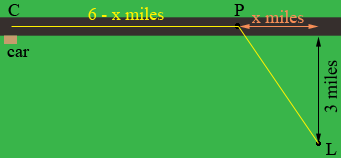SEARCH HOMEMath Central Quandaries & QueriesQuestion from Liz, a student: I am lost in the woods. I believe that I am in the woods 3 miles from a straight road. My car is located 6 miles down the road. I can walk 2miles/hour in the woods and 4 miles/hour along the road. To minimize the time needed to walk to my car, what point on the road should i walk to?Hi Liz,

Her is my diagram of the situation you describeYou are lost at $L,$ your car is at $C$ and you are going to walk through the woods to $P$ and then along the road to $C.$ It is $6 - x$ miles from $P$ to $C.$ At 4 miles per hour how long will it take yo to walk from $P$ to $C?$

Use Pythagoras' theorem to find the distance from $L$ to $P.$ At 2 miles per hour how long will it take to walk from $L$ to $P\;?$

What is the total time to walk from $L$ to $P$ and then to $C?$ Use the calculus you know to find the distance $X$ that minimizes this time.

HarleyMath Central is supported by the University of Regina and The Pacific Institute for the Mathematical Sciences.